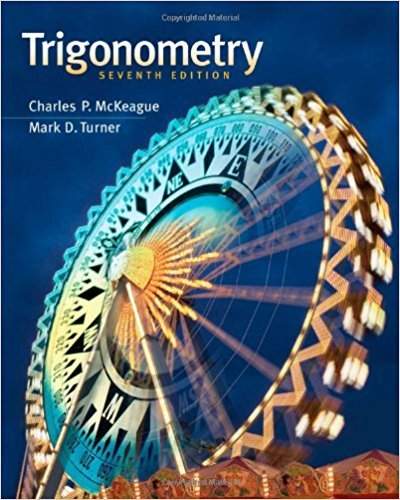×
Log in to StudySoup
Get Full Access to Trigonometry - Textbook Survival Guide
Join StudySoup for FREE
Get Full Access to Trigonometry - Textbook Survival Guide

Already have an account? Login here
×
Reset your password

# Solutions for Chapter 4.1: Basic Graphs## Full solutions for Trigonometry | 7th Edition

ISBN: 9781111826857Solutions for Chapter 4.1: Basic Graphs

Solutions for Chapter 4.1
4 5 0 364 Reviews
12
4
##### ISBN: 9781111826857

Trigonometry was written by and is associated to the ISBN: 9781111826857. This textbook survival guide was created for the textbook: Trigonometry, edition: 7. Since 86 problems in chapter 4.1: Basic Graphs have been answered, more than 41211 students have viewed full step-by-step solutions from this chapter. Chapter 4.1: Basic Graphs includes 86 full step-by-step solutions. This expansive textbook survival guide covers the following chapters and their solutions.

Key Math Terms and definitions covered in this textbook
• Block matrix.

A matrix can be partitioned into matrix blocks, by cuts between rows and/or between columns. Block multiplication ofAB is allowed if the block shapes permit.

• Cayley-Hamilton Theorem.

peA) = det(A - AI) has peA) = zero matrix.

• Circulant matrix C.

Constant diagonals wrap around as in cyclic shift S. Every circulant is Col + CIS + ... + Cn_lSn - l . Cx = convolution c * x. Eigenvectors in F.

• Cofactor Cij.

Remove row i and column j; multiply the determinant by (-I)i + j •

• Diagonalization

A = S-1 AS. A = eigenvalue matrix and S = eigenvector matrix of A. A must have n independent eigenvectors to make S invertible. All Ak = SA k S-I.

• Elimination.

A sequence of row operations that reduces A to an upper triangular U or to the reduced form R = rref(A). Then A = LU with multipliers eO in L, or P A = L U with row exchanges in P, or E A = R with an invertible E.

• Exponential eAt = I + At + (At)2 12! + ...

has derivative AeAt; eAt u(O) solves u' = Au.

• Free columns of A.

Columns without pivots; these are combinations of earlier columns.

• Incidence matrix of a directed graph.

The m by n edge-node incidence matrix has a row for each edge (node i to node j), with entries -1 and 1 in columns i and j .

• Indefinite matrix.

A symmetric matrix with eigenvalues of both signs (+ and - ).

• Independent vectors VI, .. " vk.

No combination cl VI + ... + qVk = zero vector unless all ci = O. If the v's are the columns of A, the only solution to Ax = 0 is x = o.

• Left inverse A+.

If A has full column rank n, then A+ = (AT A)-I AT has A+ A = In.

• Left nullspace N (AT).

Nullspace of AT = "left nullspace" of A because y T A = OT.

• Markov matrix M.

All mij > 0 and each column sum is 1. Largest eigenvalue A = 1. If mij > 0, the columns of Mk approach the steady state eigenvector M s = s > O.

• Pivot columns of A.

Columns that contain pivots after row reduction. These are not combinations of earlier columns. The pivot columns are a basis for the column space.

• Pivot.

The diagonal entry (first nonzero) at the time when a row is used in elimination.

• Schur complement S, D - C A -} B.

Appears in block elimination on [~ g ].

• Simplex method for linear programming.

The minimum cost vector x * is found by moving from comer to lower cost comer along the edges of the feasible set (where the constraints Ax = b and x > 0 are satisfied). Minimum cost at a comer!

• Symmetric matrix A.

The transpose is AT = A, and aU = a ji. A-I is also symmetric.

• Vector addition.

v + w = (VI + WI, ... , Vn + Wn ) = diagonal of parallelogram.